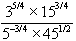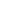The Vietnamese Mathematical Hobby Group

Math Test for Year 9/10 Students # 10

1. Factorise the following expressions:

(a)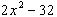(b)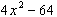(c)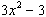(d)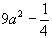(e)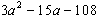(f)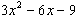(g)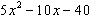2. Simplify the following:

(a)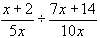(b)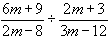(c)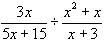(d)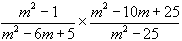(e)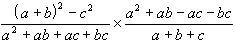(f)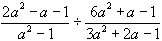3. In the triangle XYZ,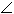X = 90o,Y = 31o, find XY.

4. Simplify the following:

(a)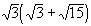(b)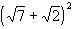(c)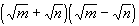5. Rationalise the denominators and hence simplify the following surds:

(a)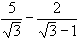(b)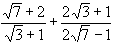(c)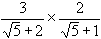6. Find the mean, median and range of the following:

(a) 4, 8, 3, 5, 1 (b) 6, 4, 9, 5, 2, 3.

7. Solve the following simultaneous equations:

(a)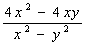(b)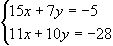(c)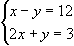8. Find the straightline equation of the following:

(a) gradient of -2 and y-intercept of 3.

(b) gradient of 1/2 and passess through (4, 5)

(c) joining two points (1, 4) and (2, 3)

(d) parallel to the line 3y = x and passes through the point (8, 0)

(e) perpendicular to the line y = 5x + 2 and passes through the origin.

9. Make the subject of the following equations as indicated:

(a) P = 2ab - 2ak [k] (b) T = a + (n - 1)d [n]

(c)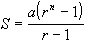[a] (d)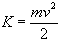[v]

10. Solve the following equations:

(a)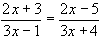(b)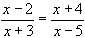(c)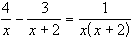(d)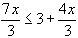(e)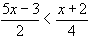(f)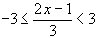Senior Students

11. Solve the following simultaneous equations:

(a)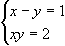(b)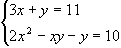(c)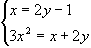12. In a triangle DABC, sinB = 4/5, a = 6, b = 9. Find sinA.

13. In a triangle DABC, A = 42o, B = 28o, BC = 6 cm. Find AB..

14. The sides of a triangular field has length 80, 90 and 100 m. Find its area.

15. Solve the following equations:

(a)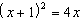(b)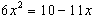(c)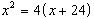16. Solve the following equation by the "complete-the-square" technique:

(a)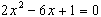(b)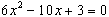17. The perimeter of a rectangle is 18 cm, its area is 20 cm2.

(a) If its length is x, express the width in terms of x

(b) Write down the area in terms of x

(c) Form a quadratic equation in x and solve it to find the length and width.

18. Given that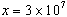and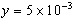. Express the following in standard notation:

(a)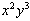(b)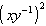19. Simplify the following: